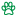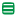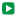Prepdog.org |      | 3rd Grade COMMON CORE STATE STANDARDS ENGLISH MATH ADVERTISEMENTLanguage             L.3.1.a - functions of nouns L.3.1.b - form and use regular and irregular plural nouns            Test 3    L.3.1.b - form and use regular and irregular plural nouns            Test 4    L.3.1.c - use abstract nouns            Test 5    L.3.1.c - use abstract nouns            Test 6    L.3.1.d - form and use regular and irregular verbs            Test 7    L.3.1.d - form and use regular and irregular verbs            Test 8    L.3.1.e - form and use the simple verb tenses            Test 9    L.3.1.e - form and use the simple verb tenses            Test 10  L.3.1.f - ensure subject-verb and pronoun antecedent agreement            Test 11  L.3.1.f - ensure subject-verb and pronoun-antecedent            Test 12  L.3.1.g - form and use comparative and superlative adjectives and adverbs            Test 13  L.3.1.g - form and use comparative and superlative adjectives and adverbs            Test 14  L.3.1.h - use coordinating and subordinating conjunctions            Test 15  L.3.1.h - use coordinating and subordinating conjunctions            Test 16  L.3.1.i - produce simple, compound, and complex sentences            Test 17  L.3.1.i - produce simple, compound, and complex sentences            Test 18  L.3.2.a capitilize appropriate words in titles            Test 19  L.3.2.a - capitalize appropriate words in titles            Test 20  L.3.2.b - use commas in addresses            Test 21  L.3.2.b - use comma in addresses            Test 22  L.3.2.c - use commas and quotation marks in dialogue            Test 23  L.3.2.d - form and use possessives            Test 24  L.3.2.d - form and use possessives            Test 25  L.3.2.e - use conventional spelling for high frequency words and for adding suffixes            Test 26  L.3.2.e - use conventional spelling for high-frequency words and for adding suffixes            Test 27  L.3.2.f - use spelling patterns and generalizations            Test 28  L.3.2.g - consult reference materials            Test 29  L.3.2.g - consult reference materials            Test 30  L.3.2.g - consult reference materials            Test 31  L.3.3.a - choose words and phrases for effect            Test 32  L.3.3.a - choose words and phrases for effect            Test 33  L.3.3.b - recognize differences between spoken-written standards of English            Test 34  L.3.3.b - recognize differences between standards of English            Test 35  L.3.4.a - use sentence level context as a word or phrase            Test 36  L.3.4.a - use sentence-level context as a clue to the meaning of a word or phrase            Test 37  L.3.4.a - use sentence-level context as a clue to the meaning of a word or phrase            Test 38  L.3.4.b - determine the meaning of a new word formed when a known affix is added            Test 39  L.3.4.b - determine the meaning of a new word formed when a known affix is added            Test 40  L.3.4.c - use a known root word as a clue to the meaning of an unknown word            Test 41  L.3.4.c - use a known root word as a clue to the meaning of an unknown word            Test 42  L.3.4.d - use glossary and dictionary to determine the meaning of words            Test 43  L.3.4.d - use glossary-dictionary to determine meaning of words            Test 44  L.3.5.a - distinguish the literal and nonliteral meanings of words            Test 45  L.3.5.a - distinguish the literal and nonliteral meanings of words            Test 46  L.3.5.b - identify real-life connections between words and their use            Test 47  L.3.5.b - identify real-life connections between words and their use            Test 48  L.3.5.c - distinquish shades of meaning among related words            Test 49  L.3.5.c - distinguish shades of meaning among related words            Test 50  L.3.6 - acquire and use accurately grade-appropirate conversational words            Test 51  L.3.6 - acquire and use accurately grade-appropriate conversational wordsReading            Test 1   3.RL.3.1 - ask and answer questions to demonstrate understanding            Test 2   3.RI.3.8 - cause and effectOperations & Algebraic Thinking 3.OA.A.1 - interpret products of whole numbers3.OA.2 Interpret whole-number quotients of whole numbers3.OA.4 determine the unknown whole number3.OA.3 determine the unknown whole number3.OA.3  use multiplication and division within 1003.OA.D.9 solve problems involving the four operations, and identify and explain patterns in arithmetic 3.OA.1 - interpret whole numbers3.OA.1 - interpret whole numbers  3.OA.2 - interpret whole number quotients3.OA.2 - interpret whole number quotients 3.OA.2 - interpret whole number quotients3.OA.3 - use multiplication and division within 1003.OA.3 - use multiplicatio and division within 1003.OA.4 - determine the unknown whole number3.OA.6 - undertand division as an unknown factor3.OA.6 - understand division as an unknown factor3.OA.8 - solve two-step word problems using the four operations3.OA.8 - solve two-step word problems using the four operations 3.OA.9 - identify arithmetic patterns3.OA.9 - identify arithmetic patterns3.OA.a - interpret whole numbers3.OA.a - interpret whole numbersNumber & Operations in Base Ten 3.NBT.A.1 - use place value understanding to round whole numbers to the nearest 10 or 1003.NBT.A.3 - multiply one-digit whole numbers by multiples of 10 in the range 10-903.NBT.2c - fluently add subtract to 1000 3.NBT.3 - multiply one digit whole numbers by multiples of 103.NBT.3 - multiply one digit whole numbers by multiples of 103.NBT.A - use place value to round whole numbers3.NBT.A.1d - use place value to round whole numbers 3.NBT - use place value to round whole numbers 3.NBT.A.1e - use place value to round whole numbers3.NBT.A.3 - multiply one digit whole numbers by multiples of 10Number & Operations: Fractions         Test 1   3.NF.A.1& 2  Understand a fraction 1/b3.NF.1        Understand fractions3.NF.1        Understand fractions 3.NF.A.3     Explain equivalence of fractions 3.NF.A.3.D  Compare two fractions3.NF.1       Understand a fraction3.NF.2.A    Represent a fraction on a number line 3.NF.2.B    Represent a fraction on a number line3.NF.2.B    Represent a fraction on a number line3.NF.3.A    Understand two fractions as equivalent3.NF.3.A    Understand two fractions as equivalent3.NF.3.B    Recognize and generate simple equivalent fractions3.NF.3.B    Recognize and generate simple equivalent fractions3.NF.3.C    Express whole numbers as fractions that are equivalent to whole numbers 3.NF.3.C    Recognize and generate simple equivalent fractions3.NF.A.3D  Compare fractionsMeasurement & Data 3.MD.A.1   Tell and write time to the nearest minute 3.MD.A.2   Measure and estiate liquid volues and masses 3.MD.B.3   Draw a scaled picture graph and a scaled bar graph to represent data   3.MD.C.6   Measure areas by counting unit suares  3.MD.7      Relate area to the operations of multiplication and addition 3.MD.C.7.  Drecognize area as additive 3.MD.D.8   Solve real world and mathematical problems involving perimeters of polygons 3.MD.1      Tell and write time to the nearest minute and measure time intervals in minutes3.MD.1      Tell and write time to the nearest minute and measure time intervals in minutes3.MD.3     Solve one and two step how many more/less problems  3.MD.4     Generate measurement data  3.MD.4     Generate measurement data  3.MD.5.b  A plane figure which can be covered by n unit squares is said to have an area of n square units 3.MD.5.b  A plane figure which can be covered by n unit squares is said to have an area of n squre units  3.MD.6 - measure areas by counting unit squares  3.MD.6 - measure areas by counting unit squares   3.MD.7.A - find the area of a rectangle with whole-number side lengths  3.MD.7.A - find the area of a rectangle with whole number side lengths 3.MD.7b - multiply side lengths to find areas of rectangles   3.MD.7.C - show the area of a rectangle with whole number side lengths   3.MD.7.C - show the area of a rectangle with whole number side lengths 3.MD.7.D - recognize area as a additive 3.MD.7.D - recognize area as additive 3.MD.8 - solve problems involving perimeters of polygons    3.MD.8 - solve problems involving perimeters of polygons   3.MD.A.1 - tell, write time to the nearest minute, measure time intervals in minutes 3.MD.A.2 - measure and estimate liquid volumes and masses of objects using standard units 3.MD.B.3d - solve one and two step how many more or less problems 3.MD.D.8 - solve problems involving perimeters of polygonsGeometry         Test 1   3.G.A.2 - Partition shapes into parts with equal areas.         Test 2   3.G.1- understand that shapes in different categories may share attributes           Test 3   3.G.1- undertand that shapes in different categories may share attributes           Test 4   3.G.2 - partition shapes into parts with equal areas          Test 5   3.G.2 - partition shapes into parts with equal areas          Test 6   3.G.A.2 - partition shapes into parts with equal areas ADVERTISEMENT Copyright © 2009- 2015 Prepdog.org, All Rights Reserved Common Core State Standards © Copyright 2010. National Governors Association Center for Best Practices and Council of Chief State School Officers. All rights reserved.